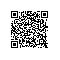MapReduce这个编程模型解决什么问题？

●  网页抓取
●  日志处理
●  索引倒排
●  查询请求汇总
●

(1)
Map函数接口：处理一个基于key/value(后简称kv)的成对(pair)数据集合，同时也输出基于kv的数据集合；
(2)
Reduce函数接口：用来合并Map输出的kv数据集合；

Map

Reduce

Map(list<pair($doc_name,$doc_content)>){

foreach(pair in list)

foreach($word in$doc_content)

echo pair($word, 1); // 输出list<k,v> } 画外音：如果有多个Map进程，输入可以是一个pair，不是一个list。 Reduce(list<pair($word, $count)>){// 大量(单词,1) map<string,int> result; foreach(pair in list) result[$word] += $count; foreach($keyin result)

echo pair($key, result[$key]); // 输出list<k,v>

}

●  并行计算
●  数据分发
●  错误处理
●  集群通讯
●使用钉钉扫一扫加入圈子
+ 订阅